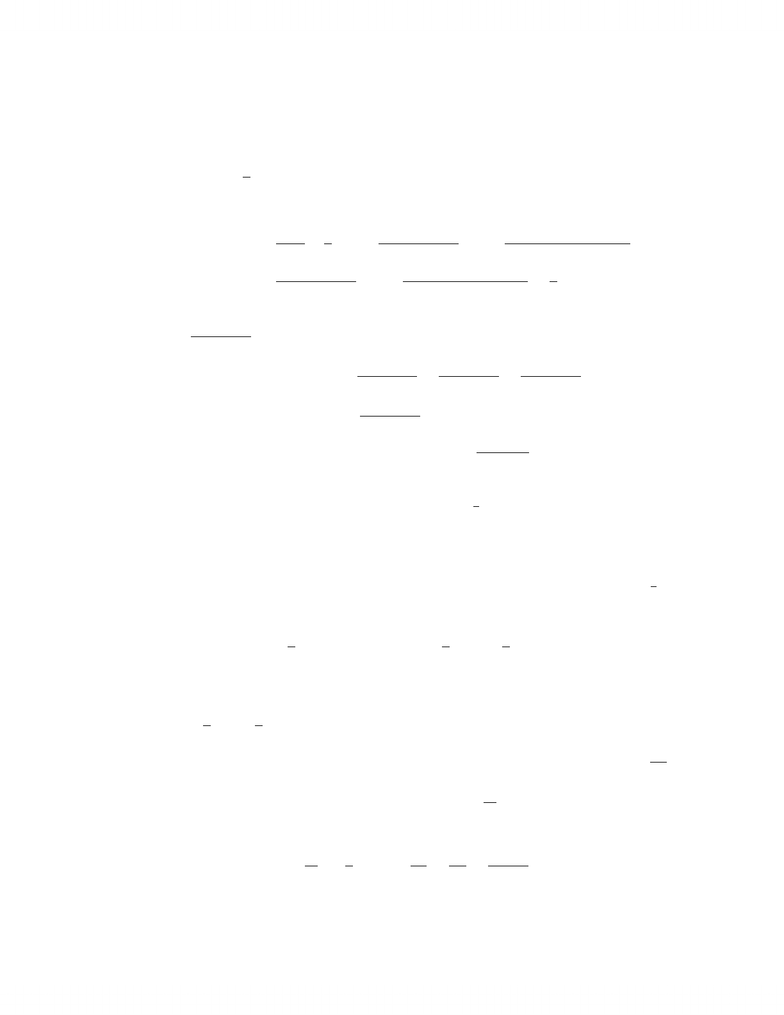# 2008 Test 2 solution

24 views4 pages
School
Department
Course
ProfessorMAT 137Y, 2008-2009 Winter Session, Term Test 2 Solutions
1. Evaluate the following limits, or show the limit does not exist.
(8%) (i) lim
x0cotx1
x.
Let Lbe the limit. Then
L=lim
h0
cosx
sinx1
x=lim
h0
xcosxsinx
xsinx
H
=lim
h0
cosxxsinxcosx
sinx+xcosx
=lim
h0xsinx
sinx+xcosx
H
=lim
h0sinxxcosx
cosx+cosxxsinx=0
2=0.
(8%) (ii) lim
x
x2+cosx
x2+2009.
Since 1cosx1, we have x21
x2+2009 x2+cosx
x2+2009 x2+1
x2+2009,
so by the squeeze theorem, lim
x
x2+cosx
x2+2009 =1.
(Note, applying L’Hˆ
opital’s Rule twice yields lim
x
2cosx
2, which does not exist, but
L’Hˆ
opital’s Rule does not apply in this situation.)
(15%) 2. Recall that the volume of a right circular cone is V=1
3πr2h, where ris the radius of the
(circular) base, and his the height of the cone. Find the largest possible volume of a right
circular cone that is inscribed in a sphere of radius R. Make sure that your answer yields an
absolute maximum.
This question is posed in SHE 4.5 #43, which provides a diagram. We maximize V=1
3πr2h.
Since r2+ (hR)2=R2by the Pythagorean Theorem, we solve for r2to get
V(h) = 1
3πR2(hR)2)h=1
3πR2h1
3π(hR)2h,
where h(0,2R). We solve for the absolute maximum; differentiating,
V0(h) = 1
3πR21
3h(hR)2+2h(hR)i=0=R2(hR)22h(hR) = 0
=R2h2+2hR R22h2+2hR =0=⇒ −3h2+4hR =0=h=4R
3.
Since lim
h0V(h) = lim
h2RV(h) = 0, the critical point h=4R
3must yield maximum volume.
Therefore the maximum volume is
V(4R
3) = 1
3πR2R2
9·4R
3=32πR3
81 .
1
Unlock document

This preview shows page 1 of the document.
Unlock all 4 pages and 3 million more documents.